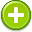#probability 中文解釋 wordnet sense Collocation Usage
Noun
/ˌpräbəˈbilətē/,Font size:probabilities, plural;
1. The extent to which something is probable; the likelihood of something happening or being the case
• - the rain will make the probability of their arrival even greater
2. A probable event
• - for a time, revolution was a strong probability
3. The most probable thing
• - the probability is that it will be phased in over a number of years
4. The extent to which an event is likely to occur, measured by the ratio of the favorable cases to the whole number of cases possible
• - the area under the curve represents probability
• - a probability of 0.5

1. a measure of how likely it is that some event will occur; a number expressing the ratio of favorable cases to the whole number of cases possible; "the probability that an unbiased coin will fall with the head up is 0.5"
2. the quality of being probable; a probable event or the most probable event; "for a while mutiny seemed a probability"; "going by past experience there was a high probability that the visitors were lost"
3. (probable) an applicant likely to be chosen
4. (probable) apparently destined; "the probable consequences of going ahead with the scheme"
5. Probability is a way of expressing knowledge or belief that an event will occur or has occurred. The concept has been given an exact mathematical meaning in probability theory, which is used extensively in such areas of study as mathematics, statistics, finance, gambling, science, and philosophy ...
6. Probabilism, in Catholic moral theology, provides a way of answering the question about what to do when one does not know what to do. Probabilism proposes that one can follow a probable opinion regarding whether an act may be performed morally, even though the opposite opinion is more probable. ...
7. the state of being probable; likelihood; an event that is likely to occur; the relative likelihood of an event happening; a number, between 0 and 1, expressing the precise likelihood of an event happening
8. (Probabilities) The range of potential future events. At any given moment, a possible future event can be described as having a certain percentage probability, e.g., a thirty-seven percent probability. As people continue to exercise their free will and make choices, that percentage can change.
9. (probabilities) [endoxa]: resting on opinion, probable, generally admitted; from doxa: expectation, notion, opinion, judgment. In both Plato and Aristotle, doxa is opposed to epistêmê, or knowledge, thus setting up a key conceptual binary in traditional Western philosophy: belief-knowledge. ...
10. (PROBABLE) CAUSE:  A reasonable belief that a crime was committed, and the person accused of the crime was responsible. A probable cause hearing is held in felony matters.
11. (Probable) Something that is likely to exist, occur, or be true, although evidence is insufficient to prove or predict it with certainty.
12. (Probable) evidence indicates there is data strong enough to support a probable causal relationship between the exposure and the risk of a particular cancer. There is enough evidence to generally justify recommendations designed to reduce the risk of cancer.
13. (probable) Lone Horn, Minneconjou chief (d. 1875)
14. (probable) happening more often than not
15. The relative likelihood of a particular outcome among all possible outcomes.
16. A chance, or likelihood, that a certain event might happen.
17. The likelihood that an event will occur.
18. A branch of mathematics that measures the likelihood that an event will occur.  Probabilities are expressed as numbers between 0 and 1.  The probability of an impossible event is 0, while an event that is certain to occur has a probability of 1.
19. A description of the likely occurrence of a particular event. Probability is conventionally expressed on a scale from 0 to 1; a rare event has a probability close to 0, a very common event has a probability close to 1.
20. For an experiment, the total number of successful events divided by the total number of possible events.
21. The chance that an event occurs. It is usually expressed as a percent. Zero percent denotes impossibility, while 100% denotes certainty.
22. The chance that a phenomenon has a of occurring randomly. As a statistical measure, it shown as p (the "p" factor).
23. A probability assignment is a numerical encoding of the relative state of knowledge.
24. The likelihood that results in a test were due to chance. See also significance.
25. The extent to which something is likely to happen or to be the case.